### HARRY PooterHover over the thumbnail for a full-size version.

Author espada777777 author:espada777777 bitesized harry harryandthepotter harrypotter n-art rated 2008-03-01 4 by 35 people. \$HARRY Pooter#espada777777#none#00000000000000000000000000000000000000000000000000000000000000000000000000000000000000000000000000000000000000000000000000000000000000000000000000000000000000000000000000000000000000000000000000000000000000000000000000000000000000000000000000000000000000000000000000000000000000000000000000000000000000000000000000000000000000000000000000000000000000000000000000000000000000000000000000000000000000000000000000000000000000000000000000000000000000000000000000000000000000000000000000000000000000000000000000000000000000000000000000000000000000000000000000000000000000000000000000000000000000000000000000000000000000000000000000000000000000000000000000000000000000000000000000000000000000000000000000000000000000000|12^222,307!12^222,307!12^222,310!12^223,317!12^226,324!12^231,332!12^237,339!12^237,338!12^239,342!12^243,345!12^247,350!12^252,359!12^261,366!12^267,372!12^274,378!12^284,383!12^292,387!12^302,391!12^311,392!12^322,390!12^331,390!12^343,391!12^350,394!12^357,400!12^370,401!12^381,404!12^389,404!12^404,403!12^426,401!12^429,400!12^430,399!12^433,397!12^439,394!12^447,391!12^453,389!12^463,390!12^474,390!12^486,388!12^499,383!12^517,377!12^525,372!12^533,369!12^540,365!12^548,361!12^556,354!12^567,345!12^574,336!12^579,325!12^581,317!12^589,307!12^597,300!12^615,291!12^628,279!12^632,271!12^635,264!12^635,263!12^635,263!12^623,284!12^614,294!12^598,302!12^226,306!12^226,306!12^235,312!12^242,318!12^248,323!12^257,330!12^273,338!12^285,343!12^303,345!12^317,346!12^332,340!12^324,343!12^297,345!12^270,339!12^263,335!12^294,341!12^312,342!12^326,340!12^337,335!12^349,332!12^356,331!12^364,330!12^386,330!12^395,330!12^392,330!12^371,329!12^385,330!12^419,332!12^440,328!12^441,327!12^382,334!12^423,334!12^446,331!12^447,330!12^404,331!12^432,330!12^448,325!12^472,317!12^487,310!12^504,303!12^459,322!12^454,326!12^488,306!12^495,305!12^514,302!12^528,299!12^548,292!12^564,289!12^576,286!12^585,283!12^539,298!12^551,294!12^594,284!12^596,283!12^616,280!12^619,278!12^593,285!12^601,283!12^623,275!12^627,272!12^599,285!12^604,283!12^623,251!12^627,250!12^633,247!12^642,247!12^647,249!12^651,261!12^651,257!12^647,245!12^621,250!12^640,248!12^651,248!12^652,254!12^645,249!12^652,265!12^653,279!12^654,260!12^653,258!12^655,276!12^655,280!12^655,262!12^205,313!12^206,306!12^210,295!12^233,292!12^230,291!12^217,291!12^207,301!12^205,309!12^212,299!12^217,293!12^228,298!12^222,291!12^222,291!12^222,291!12^276,346!12^276,348!12^277,351!12^278,361!12^278,362!12^289,364!12^295,364!12^299,364!12^301,357!12^301,348!12^301,348!12^301,365!12^285,364!12^303,366!12^309,364!12^320,361!12^312,364!12^310,365!12^321,363!12^324,357!12^324,355!12^324,345!12^324,349!12^325,353!12^335,351!12^323,356!12^336,351!12^339,351!12^344,349!12^346,342!12^346,338!12^345,353!12^327,355!12^347,356!12^347,359!12^347,362!12^347,365!12^355,364!12^362,364!12^369,363!12^369,357!12^369,342!12^369,333!12^368,345!12^368,358!12^371,357!12^369,347!12^371,355!12^375,352!12^381,352!12^385,352!12^374,352!12^378,351!12^384,348!12^384,337!12^386,348!12^386,349!12^393,348!12^390,348!12^390,346!12^407,347!12^406,348!12^393,348!12^401,348!12^406,343!12^406,337!12^406,344!12^408,350!12^411,355!12^408,353!12^412,358!12^418,365!12^425,361!12^433,361!12^420,365!12^426,365!12^431,360!12^430,354!12^430,349!12^429,344!12^427,342!12^397,334!12^403,334!12^415,334!12^426,346!12^445,345!12^437,345!12^435,345!12^441,345!12^447,345!12^448,332!12^448,345!12^449,334!12^449,334!12^460,334!12^454,336!12^463,334!12^466,335!12^460,337!12^473,332!12^475,333!12^475,322!12^475,322!12^473,332!12^448,338!12^448,346!12^448,346!12^477,320!12^477,331!12^478,339!12^478,341!12^478,344!12^483,344!12^482,343!12^487,342!12^493,338!12^492,314!12^492,339!12^491,333!12^491,319!12^491,314!12^491,318!12^491,336!12^494,330!12^490,326!12^494,326!12^474,317!12^481,311!12^484,308!12^463,321!12^463,321!12^495,329!12^498,330!12^500,330!12^503,330!12^496,330!12^508,327!12^509,323!12^509,315!12^509,311!12^508,317!12^508,311!12^271,351!12^269,350!12^264,350!12^261,350!12^260,342!12^259,338!12^258,329!12^258,342!12^254,340!12^251,340!12^246,339!12^240,338!12^238,329!12^239,336!12^240,327!12^240,316!12^238,316!12^230,317!12^239,330!12^244,321!12^514,315!12^515,315!12^525,315!12^522,315!12^512,317!12^520,317!12^525,317!12^526,308!12^524,308!12^524,307!12^524,307!12^524,307!12^524,319!12^525,325!12^525,327!12^532,329!12^532,329!12^523,329!12^535,329!12^540,329!12^542,327!12^542,313!12^542,310!12^543,302!12^543,303!12^541,326!12^541,329!12^541,318!12^541,316!12^549,320!12^550,315!12^557,314!12^561,314!12^561,315!12^561,304!12^563,287!12^563,298!12^561,315!12^560,302!12^561,302!12^568,308!12^568,306!12^579,304!12^581,304!12^582,294!12^583,288!12^583,300!12^583,300!12^591,295!12^593,293!12^602,294!12^599,291!12^568,290!12^556,292!12^542,297!12^532,300!12^526,300!12^514,302!12^496,306!12^489,306!12^473,314!12^469,318!12^280,342!12^289,343!12^302,344!12^314,348!12^329,345!12^337,338!12^350,333!12^359,331!12^373,331!12^386,331!12^407,332!12^415,334!12^357,392!12^360,391!12^364,390!12^371,388!12^382,386!12^390,385!12^404,384!12^419,384!12^425,384!12^406,384!12^396,384!12^398,385!12^419,387!12^427,387!12^435,387!12^436,388!12^406,389!12^377,392!12^403,389!12^398,392!12^392,399!12^398,393!12^406,388!12^357,394!12^369,400!12^386,404!12^395,407!12^411,403!12^423,400!12^427,399!12^403,403!12^387,402!12^368,398!12^350,396!12^376,285!12^370,285!12^367,283!12^366,280!12^368,277!12^372,275!12^378,274!12^381,274!12^385,275!12^385,276!12^385,281!12^378,283!12^378,284!12^381,285!12^240,347!12^250,361!12^258,364!12^268,373!12^280,379!12^298,389!12^314,389!12^326,390!12^302,390!12^458,390!12^330,386!12^336,393!12^342,393!12^347,395!12^353,398!12^360,403!12^379,407!12^386,407!12^394,407!12^410,406!12^378,408!12^369,404!12^377,404!12^407,408!12^426,408!12^431,405!12^442,402!12^447,400!12^460,398!12^466,397!12^443,398!12^456,392!12^472,391!12^459,396!12^469,396!12^487,395!12^502,390!12^512,384!12^527,380!12^539,374!12^548,363!12^564,348!12^571,338!12^577,323!12^580,315!12^545,358!12^528,369!12^493,381!12^482,382!12^468,383!12^423,273!12^417,274!12^417,274!12^421,274!12^429,271!12^438,270!12^439,274!12^437,279!12^428,282!12^419,278!12^419,278!12^424,284!12^430,284!12^435,284!12^434,276!12^351,270!12^352,270!12^353,268!12^354,265!12^358,261!12^362,259!12^368,258!12^354,261!12^437,252!12^441,252!12^447,253!12^453,255!12^454,259!12^454,261!12^449,255!12^439,252!12^388,247!12^390,244!12^395,242!12^403,241!12^413,242!12^419,250!12^413,247!12^402,246!12^395,246!12^388,249!12^397,245!12^377,244!12^381,240!12^385,233!12^387,219!12^388,210!12^388,206!12^388,227!12^383,238!12^377,248!12^372,250!12^435,244!12^429,239!12^427,236!12^424,226!12^423,209!12^423,212!12^425,230!12^429,242!12^436,243!12^424,230!12^422,225!12^422,214!12^392,250!12^388,253!12^385,258!12^384,258!12^392,253!12^403,247!12^424,255!12^500,130!12^494,130!12^486,132!12^478,133!12^473,133!12^467,131!12^463,127!12^459,122!12^458,112!12^458,109!12^462,107!12^468,105!12^477,102!12^484,102!12^490,106!12^501,112!12^503,119!12^503,127!12^503,128!12^473,106!12^473,108!12^476,114!12^480,115!12^482,115!12^487,113!12^492,109!12^491,108!12^476,113!12^479,110!12^478,109!12^481,109!12^483,108!12^302,161!12^294,153!12^295,142!12^302,137!12^315,140!12^319,155!12^310,161!12^297,156!12^295,145!12^299,140!12^307,140!12^315,144!12^315,156!12^306,160!12^320,149!12^300,143!12^303,146!12^306,150!12^310,145!12^309,145!12^304,146!12^461,88!12^458,90!12^451,93!12^449,95!12^447,98!12^446,105!12^445,113!12^445,120!12^451,131!12^453,135!12^457,139!12^466,144!12^474,147!12^483,148!12^491,148!12^498,144!12^501,143!12^505,140!12^512,137!12^517,132!12^520,124!12^521,113!12^521,108!12^514,96!12^508,91!12^501,85!12^497,84!12^490,84!12^480,84!12^468,86!12^475,86!12^487,87!12^504,88!12^517,93!12^519,101!12^521,108!12^510,131!12^504,140!12^487,143!12^470,143!12^457,137!12^448,130!12^445,125!12^447,128!12^450,135!12^452,141!12^453,144!12^455,149!12^464,153!12^469,154!12^472,155!12^478,157!12^484,159!12^486,159!12^493,159!12^499,156!12^505,155!12^508,153!12^512,150!12^516,148!12^520,144!12^523,141!12^525,131!12^525,129!12^525,119!12^524,114!12^523,131!12^456,102!12^458,99!12^473,96!12^459,93!12^483,96!12^500,93!12^494,97!12^466,93!12^459,95!12^490,95!12^504,99!12^441,117!12^440,117!12^439,115!12^428,114!12^425,113!12^418,112!12^413,112!12^406,112!12^398,112!12^396,112!12^389,113!12^383,114!12^379,116!12^374,118!12^366,120!12^361,123!12^359,124!12^349,129!12^348,131!12^357,127!12^330,133!12^327,128!12^319,120!12^317,119!12^308,119!12^305,119!12^297,123!12^290,126!12^286,130!12^281,137!12^279,144!12^279,151!12^281,163!12^283,171!12^289,175!12^292,180!12^306,181!12^314,180!12^324,176!12^329,171!12^331,164!12^336,153!12^336,145!12^331,138!12^334,153!12^333,163!12^320,176!12^295,175!12^275,156!12^279,168!12^283,172!12^283,180!12^283,183!12^283,190!12^290,195!12^295,196!12^305,196!12^313,196!12^323,192!12^332,185!12^337,176!12^339,156!12^338,149!12^338,161!12^281,184!12^276,184!12^275,172!12^274,166!12^276,154!12^349,130!12^347,133!12^339,143!12^337,147!12^343,136!12^346,132!12^338,165!12^337,174!12^322,191!12^317,192!12^308,193!12^285,115!12^276,120!12^278,117!12^290,110!12^304,109!12^317,109!12^306,107!12^285,108!12^276,119!12^283,114!12^298,110!12^573,112!12^577,106!12^582,103!12^591,101!12^599,110!12^600,123!12^599,142!12^602,119!12^598,112!12^585,101!12^588,103!12^601,108!12^600,136!12^600,143!12^602,153!12^602,162!12^602,168!12^599,170!12^592,174!12^587,173!12^585,173!12^590,173!12^581,124!12^583,121!12^590,119!12^591,119!12^592,132!12^592,141!12^577,153!12^582,153!12^586,153!12^591,159!12^582,141!12^584,140!12^590,142!12^526,111!12^530,110!12^534,108!12^530,111!12^536,111!12^547,106!12^558,105!12^538,109!12^554,107!12^561,106!12^569,105!12^573,105!12^578,105!12^554,107!12^566,102!12^576,100!12^577,100!12^250,168!12^247,166!12^245,163!12^241,163!12^238,165!12^239,187!12^239,190!12^239,200!12^239,213!12^239,206!12^237,195!12^236,178!12^236,170!12^237,188!12^239,206!12^242,210!12^248,206!12^250,205!12^251,204!12^247,179!12^244,179!12^243,189!12^244,194!12^249,197!12^278,158!12^271,156!12^262,151!12^262,151!12^247,153!12^243,155!12^242,156!12^243,155!12^253,151!12^258,150!12^270,153!12^275,153!12^390,428!12^405,427!12^410,426!12^426,428!12^402,429!12^399,429!12^409,430!12^430,426!12^441,427!12^432,428!12^409,429!12^409,429!12^423,427!12^410,48!12^410,50!12^408,54!12^407,58!12^406,62!12^403,71!12^403,72!12^409,70!12^409,70!12^403,71!12^411,70!12^414,68!12^420,68!12^422,68!12^418,74!12^418,77!12^416,90!12^416,90!12^416,84!12^416,88!12^416,92!12^366,40!12^359,42!12^354,59!12^352,71!12^354,65!12^360,46!12^368,40!12^358,56!12^355,72!12^364,34!12^351,46!12^343,60!12^332,82!12^338,64!12^352,46!12^362,41!12^354,49!12^341,69!12^336,84!12^340,59!12^356,42!12^340,67!12^334,86!12^335,56!12^342,41!12^335,50!12^325,70!12^320,81!12^320,81!12^332,41!12^343,38!12^345,38!12^322,72!12^320,79!12^328,54!12^339,42!12^340,42!12^322,72!12^323,68!12^347,35!12^349,35!12^354,35!12^355,37!12^316,57!12^312,68!12^301,88!12^313,52!12^341,34!12^322,47!12^306,72!12^303,81!12^318,52!12^340,36!12^322,52!12^296,77!12^330,52!12^326,53!12^299,66!12^285,77!12^337,47!12^313,52!12^301,56!12^288,59!12^280,60!12^267,62!12^261,64!12^272,59!12^282,56!12^293,51!12^301,47!12^309,40!12^318,35!12^326,27!12^328,26!12^320,30!12^300,46!12^286,54!12^274,59!12^261,63!12^256,65!12^250,74!12^242,86!12^238,95!12^250,75!12^254,70!12^244,87!12^242,99!12^238,112!12^269,65!12^263,75!12^262,81!12^262,104!12^304,52!12^300,64!12^290,86!12^289,90!12^308,57!12^321,40!12^307,58!12^301,76!12^300,64!12^304,50!12^271,91!12^271,91!12^294,66!12^268,96!12^265,105!12^275,75!12^260,109!12^258,126!12^255,139!12^255,143!12^251,105!12^253,100!12^246,99!12^246,113!12^244,132!12^244,138!12^248,83!12^241,112!12^249,111!12^262,73!12^263,80!12^255,110!12^264,77!12^266,71!12^265,64!12^250,98!12^259,79!12^261,76!12^258,95!12^254,85!12^254,91!12^258,90!12^258,91!12^255,112!12^254,121!12^250,131!12^246,145!12^246,150!12^242,120!12^251,97!12^249,132!12^247,138!12^257,69!12^276,67!12^269,105!12^272,82!12^277,79!12^280,90!12^287,67!12^287,60!12^295,69!12^311,77!12^320,68!12^324,47!12^351,60!12^341,69!12^331,61!12^328,47!12^337,28!12^352,39!12^366,30!12^368,30!12^381,31!12^365,38!12^364,41!12^359,61!12^363,40!12^361,74!12^361,78!12^392,32!12^415,28!12^428,40!12^435,50!12^436,54!12^424,35!12^412,33!12^397,28!12^394,28!12^415,31!12^387,23!12^363,53!12^353,78!12^353,79!12^366,41!12^366,43!12^359,83!12^359,92!12^353,73!12^347,58!12^343,51!12^335,33!12^325,38!12^328,36!12^349,23!12^364,31!12^357,34!12^348,35!12^364,33!12^390,28!12^402,27!12^417,28!12^374,29!12^356,24!12^371,28!12^424,31!12^433,42!12^441,51!12^444,60!12^443,55!12^454,56!12^454,55!12^444,63!12^451,60!12^461,60!12^467,59!12^479,59!12^480,59!12^483,59!12^489,59!12^485,60!12^471,60!12^481,59!12^491,59!12^494,57!12^505,57!12^507,52!12^510,58!12^520,59!12^510,57!12^517,54!12^524,43!12^533,56!12^534,41!12^530,52!12^534,54!12^537,50!12^541,49!12^549,56!12^551,61!12^546,50!12^553,61!12^557,67!12^568,84!12^559,69!12^557,68!12^563,78!12^565,81!12^571,88!12^573,91!12^576,94!12^586,91!12^592,98!12^592,88!12^589,82!12^585,75!12^585,76!12^590,86!12^586,73!12^584,69!12^580,61!12^578,59!12^575,54!12^574,53!12^570,47!12^568,43!12^561,36!12^554,31!12^550,24!12^552,25!12^564,36!12^434,33!12^434,33!12^447,46!12^426,26!12^437,35!12^448,45!12^454,52!12^437,43!12^445,45!12^454,52!12^458,44!12^448,40!12^443,32!12^442,28!12^455,45!12^460,49!12^471,51!12^458,43!12^453,37!12^453,29!12^451,25!12^461,33!12^469,42!12^473,46!12^478,49!12^469,37!12^466,30!12^466,27!12^475,38!12^480,48!12^494,55!12^349,84!12^340,85!12^327,84!12^318,83!12^306,82!12^297,82!12^291,84!12^282,91!12^266,100!12^255,109!12^250,115!12^281,91!12^320,81!12^344,84!12^353,73!12^349,62!12^339,62!12^339,77!12^336,80!12^485,46!12^478,52!12^482,51!12^486,40!12^473,33!12^473,23!12^344,30!12^346,29!12^487,31!12^474,30!12^452,25!12^428,24!12^434,26!12^458,27!12^488,26!12^496,30!12^497,41!12^498,48!12^492,50!12^483,32!12^499,35!12^505,33!12^512,54!12^512,41!12^507,42!12^509,50!12^508,43!12^510,44!12^520,31!12^508,31!12^512,27!12^517,36!12^525,45!12^523,47!12^519,51!12^525,48!12^533,44!12^542,33!12^533,31!12^529,27!12^529,27!12^531,30!12^540,38!12^545,43!12^559,50!12^561,63!12^564,76!12^573,79!12^579,86!12^580,88!12^572,72!12^557,40!12^555,39!12^566,60!12^573,67!12^254,115!12^247,129!12^243,128!12^240,105!12^241,97!12^245,90!12^252,84!12^250,100!12^246,128!12^242,215!12^244,211!12^245,217!12^244,222!12^245,228!12^241,217!12^242,220!12^248,234!12^248,240!12^244,253!12^242,232!12^243,232!12^246,244!12^246,253!12^245,254!12^243,263!12^242,273!12^239,276!12^239,276!12^239,267!12^243,266!12^243,271!12^241,275!12^234,274!12^230,272!12^227,272!12^218,272!12^213,275!12^207,279!12^205,280!12^202,287!12^196,299!12^195,302!12^193,310!12^192,320!12^190,316!12^193,298!12^195,309!12^195,295!12^201,286!12^197,291!12^194,305!12^193,316!12^193,329!12^193,333!12^196,341!12^200,346!12^193,337!12^190,332!12^196,340!12^201,350!12^205,358!12^208,362!12^212,363!12^216,364!12^202,357!12^198,354!12^208,364!12^207,369!12^207,375!12^208,382!12^212,392!12^217,395!12^223,397!12^215,398!12^220,399!12^250,406!12^235,404!12^224,398!12^225,398!12^236,405!12^242,407!12^249,408!12^234,403!12^229,403!12^234,413!12^235,417!12^236,425!12^242,436!12^251,445!12^249,443!12^249,443!12^236,405!12^240,428!12^243,442!12^247,449!12^259,452!12^251,450!12^259,454!12^267,457!12^275,459!12^282,461!12^287,462!12^293,463!12^306,464!12^311,464!12^291,462!12^289,461!12^267,462!12^265,466!12^265,473!12^265,477!12^265,483!12^271,498!12^291,510!12^278,502!12^272,494!12^268,490!12^273,502!12^279,510!12^285,516!12^292,520!12^303,521!12^310,523!12^319,526!12^293,516!12^272,503!12^270,506!12^269,510!12^266,512!12^260,517!12^249,526!12^248,528!12^248,532!12^249,539!12^255,545!12^264,551!12^268,555!12^271,558!12^263,552!12^251,541!12^245,531!12^245,531!12^220,535!12^241,538!12^236,536!12^232,535!12^219,538!12^213,541!12^206,546!12^205,558!12^216,571!12^212,568!12^209,554!12^208,550!12^211,555!12^221,571!12^207,556!12^198,559!12^194,562!12^186,567!12^183,572!12^183,572!12^594,177!12^591,188!12^592,191!12^595,198!12^598,213!12^606,228!12^605,223!12^600,206!12^592,186!12^597,218!12^598,219!12^605,229!12^612,231!12^617,232!12^615,232!12^620,232!12^626,232!12^629,236!12^633,241!12^638,244!12^657,267!12^656,273!12^656,276!12^660,275!12^665,285!12^668,289!12^665,295!12^661,297!12^657,300!12^648,307!12^646,314!12^642,326!12^642,326!12^653,309!12^658,302!12^671,287!12^664,302!12^654,313!12^648,318!12^640,323!12^637,327!12^636,330!12^627,342!12^627,342!12^613,348!12^615,348!12^621,350!12^636,335!12^637,329!12^638,324!12^628,343!12^628,343!12^633,348!12^634,356!12^631,365!12^612,382!12^612,382!12^627,374!12^629,370!12^613,383!12^601,390!12^597,393!12^590,396!12^585,397!12^587,398!12^615,384!12^622,381!12^624,376!12^623,392!12^624,395!12^613,391!12^623,405!12^623,416!12^621,429!12^610,428!12^592,433!12^574,443!12^587,441!12^596,435!12^615,423!12^621,416!12^614,427!12^598,433!12^586,439!12^578,440!12^564,447!12^403,467!12^413,467!12^425,467!12^440,468!12^454,468!12^469,467!12^443,470!12^429,471!12^407,467!12^404,469!12^433,471!12^459,467!12^616,430!12^619,431!12^621,434!12^624,446!12^623,456!12^621,466!12^620,472!12^615,483!12^602,492!12^590,501!12^574,506!12^556,510!12^548,513!12^400,523!12^414,525!12^428,526!12^446,526!12^459,523!12^442,525!12^427,525!12^412,523!12^406,523!12^408,524!12^432,528!12^445,527!12^614,492!12^619,492!12^629,498!12^639,511!12^619,505!12^632,515!12^628,510!12^640,521!12^650,530!12^641,521!12^630,502!12^619,495!12^634,507!12^652,535!12^651,529!12^655,543!12^654,551!12^650,564!12^645,569!12^652,548!12^655,546!12^656,545!12^668,548!12^671,549!12^680,553!12^693,560!12^699,567!12^700,572!12^704,570!12^710,571!12^717,576!12^111,92!12^107,92!12^98,95!12^95,99!12^87,104!12^83,109!12^82,112!12^77,124!12^76,133!12^76,142!12^78,154!12^86,164!12^92,170!12^103,172!12^110,173!12^115,174!12^122,174!12^131,169!12^136,167!12^140,163!12^136,163!12^127,165!12^121,161!12^119,160!12^109,155!12^106,152!12^99,146!12^98,139!12^98,133!12^98,127!12^101,120!12^112,114!12^114,113!12^121,109!12^130,109!12^141,113!12^134,100!12^126,93!12^121,91!12^107,94# This is harry after he realized killing ron and hermone was wrong

## Other maps by this author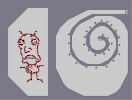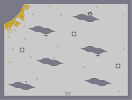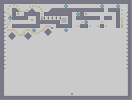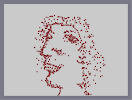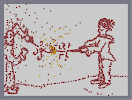RACE ART COMBO PACK DELUXE LASER CLOUDS The Arter's Incision Harry Potter The most beautiful girl ever HARRY potter

## Comments

Pages: (0)

hilarious

### ...

...
...
...
so awesome
...
cant appreciate it enough!
...
...

### sorry for the comment spam

but this may very well be the ultimate source of happiness and all that is good in life :P

### this was discusting.

and then i read the comment.
then it was hilarious.
5/5 soooooo wish i could favorite this. sooooo much. aaaaah!

### Uhhhh...

This is freaky...I give this a 4, -1/2 because it freakes me out...

FUNNY AS HELL!

### NO!

IT'S HARRY THE HUTT!
IT'S JABBA THE POTTER!

JABBA THE HARRY!

### Lol that this got bitesized

I was the first to rate this I believe, maybe second, and I fived.
Why? Because it made me laugh. (Also, I knew so many people would rate it down my 5 wouldn't actually contribute to it having a GOOD rating. However, these Espada HP maps make me laugh, each one of them, because the art is good and yet so hideous, and the descriptions are absolutely ludicrous. However, don't just blatantly criticize this guy, these narts are funny and obviously meant to be a joke.
/rant

5/5

jabba.

LOL!!!
ROFL!!!

### hahaha

thats pretty funny. XD

### Haha

tawphyt just defended harry potter and took this seriously

nice nart

good job

I love it!

Thats hilarious.

5/5

### ALL OF YOU STOP GETTING YOUR PANTIES IN A FUCKING BUNCH.

Except for T3chno, becasue he's not freaking out.

Take a joke you hard-asses.

### take

a joke, and it's not a bad art anyways.

### Lol

He looks like Jabba the Hut.

### By the way...

You keep spelling 'hermone' wrong. It's HERMIONE.

### Stop!

Stop making fun of J.K. Rowling and her books & characters!

wow...

wow...

Ahahahahah.

4 pres !

You own!